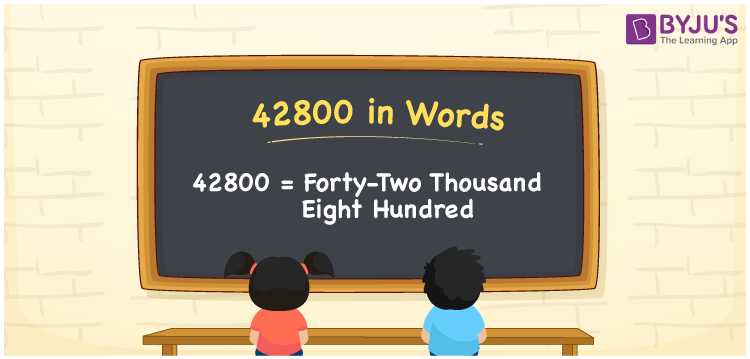# 42800 in words

42800 in words is written as Forty Two Thousand Eight Hundred. In 42800, 4 has a place value of ten thousand, 2 is in the place value of thousand and 8 is in the place value of hundred. The article on Place Value gives more information. The number 42800 is used in expressions that relate to money, distance, Social media views, and many more. For example, “A new model of mobile costs Forty Two Thousand Eight Hundred Rupees”. Another example, “Today’s total pandemic cases rose to Forty Two Thousand Eight Hundred”.

 42800 in words Forty Two Thousand Eight Hundred Forty Two Thousand Eight Hundred in Numbers 42800

## 42800 in English Words## How to Write 42800 in Words?

We can convert 42800 to words using a place value chart. The number 42800 has 5 digits, so let’s make a chart that shows the place value up to 5 digits.

 Ten thousand Thousands Hundreds Tens Ones 4 2 8 0 0

Thus, we can write the expanded form as:

4 × Ten thousand + 2 × Thousand + 8 × Hundred + 0 × Ten + 0 × One

= 4 × 10000 + 2 × 1000 + 8 × 100 + 0 × 10 + 0 × 1

= 42800.

= Forty Two Thousand Eight Hundred.

42800 is the natural number that is succeeded by 42799 and preceded by 42801.

42800 in words – Forty Two Thousand Eight Hundred.

Is 42800 an odd number? – No.

Is 42800 an even number? – Yes.

Is 42800 a perfect square number? – No.

Is 42800 a perfect cube number? – No.

Is 42800 a prime number? – No.

Is 42800 a composite number? – Yes.

## Solved Example

1. Write the number 42800 in expanded form

Solution: 4 x 10000 + 2 x 1000 + 8 x 100 + 0 x 10 + 0 x 1

Or Just 4 x 10000 + 2 x 1000 + 8 x 100

We can write 42800 = 40000 + 2000 + 800 + 0 + 0

= 4 x 10000 + 2 x 1000 + 8 x 100 + 0 x 10 + 0 x 1.

## Frequently Asked Questions on 42800 in words

Q1

### How to write the number 42800 in words?

42800 in words is written as Forty Two Thousand Eight Hundred.
Q2

### State whether True or False. 42800 is divisible by 3?

False. 42800 is not divisible by 3.
Q3

### Is 42800 divisible by 10?

Yes. 42800 is divisible by 10.